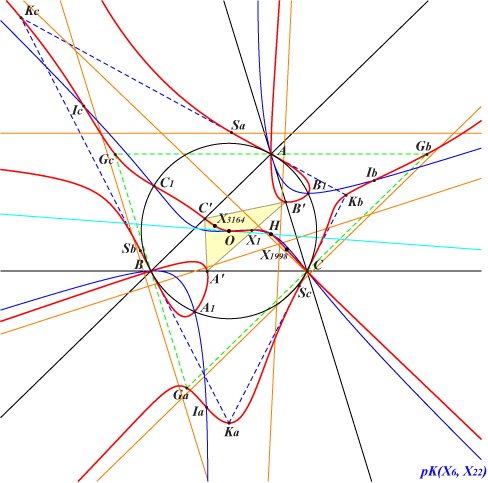too complicated to be written here. Click on the link to download a text file.X(1), X(3), X(4), X(1988), X(3164), X(5374) Ia, Ib, Ic : excenters Ga, Gb, Gc : vertices of the antimedial triangle Ka, Kb, Kc : vertices of the tangential triangle A', B', C' : vertices of the cevian triangle of O other points described belowQ103 is the locus of point P such that the cevian triangles of P and its isogonal conjugate P* are orthologic. Q103 is an isogonal circum-sextic with nodes A, B, C. The nodal tangents are the altitudes of ABC and the sidelines of the tangential triangle KaKbKc. Q103 meets KbKc at A (thrice), Kb, Kc, and a sixth point Sa on the line GaA' which contains X(3164) since it is the X(2) Ceva congugate of X(3). Sb and Sc are defined likewise and Q103 also contains their isogonal conjugates Sa*, Sb*, Sc* on the sidelines of GaGbGc. Q103 has six asymptotes : three are parallel to the sidelines of ABC and three are parallel to those of the cubic pK(X6, X22), a member of the Euler pencil. Q103 also contains A1, B1, C1 which are the isogonal conjugates of the infinite points of this latter cubic. *** Related loci : K003 is the locus of point P such that the pedal triangles of P and its isogonal conjugate are orthologic. K007 is the locus of point P such that the cevian triangles of P and its isotomic conjugate are orthologic. K146 is the locus of point P such that the pedal triangles of P and its isotomic conjugate are orthologic. K024 is the locus of point P such that the pedal triangles of P and its isogonal conjugate are parallelogic. K149 is the locus of point P such that the pedal triangles of P and its isotomic conjugate are parallelogic. The locus of point P such that the cevian triangles of P and its isogonal conjugate are parallelogic is another sextic comparable to Q103. The locus of point P such that the cevian triangles of P and its isotomic conjugate are parallelogic is a Tucker cubic, the locus of points P such that the area of the cevian triangle of P is half that of ABC. *** Generalization : If P* is the image of P under the isoconjugation with pole Ω then : • the locus of point P such that the pedal triangles of P and P* are orthologic is pK(Ω, O) together with some trivial curves. See CL021. • the locus of point P such that the pedal triangles of P and P* are parallelogic is nK0(Ω, K) together with some trivial curves. See CL022. The corresponding loci related to cevian triangles are sextics.